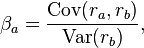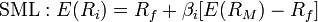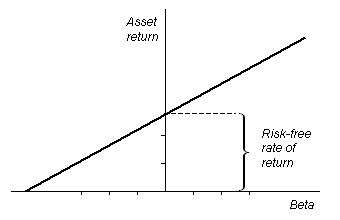## Expected Risk and Risk Premium

Overall riskiness of an asset is composed of its own individual risk (beta) along with its risk in relation to the market as a whole.

### Learning Objectives

Use a stock’s beta to estimate a stock’s daily growth or decline.

### Key Takeaways

#### Key Points

• In return for undertaking risk, investors expect to be compensated in such as a way as to reasonably reward them.
• Systemic risk is the risk associated with an entire financial system or entire market. It cannot be diversified away.
• Unsystematic risk is risk to which only specific classes of securities or industries are vulnerable, and with proper grouping of assets it can be reduced or even eliminated.
• Beta is a number describing the correlated volatility of an asset in relation to the volatility of the benchmark that said asset is being compared to — usually the market as expressed in an index.
• The term risk premium refers to the amount by which an asset’s expected rate of return exceeds the risk-free interest rate.

#### Key Terms

• Risk free rate: Risk-free interest rate is the theoretical rate of return of an investment with no risk of financial loss.
• treasury bill: Treasury bills (or T-Bills) mature in one year or less. They do not pay interest prior to maturity; instead they are sold at a discount of the par value to create a positive yield to maturity.

A certain amount of risk is inherent in any investment. Risk can be defined, generally, as the potential that a chosen action or activity (including the choice of inaction) will lead to a loss or an undesirable outcome. The notion of risk implies that a choice having an influence on the outcome exists. More specifically to finance, risk can be seen as relating to the probability of uncertain future events. In return for undertaking risk, investors expect to be compensated in such as a way as to reasonably reward them. This is a central them in the subject of finance. In the financial realm, two types of risk exist: systematic and unsystematic.

Systemic risk is the risk associated with an entire financial system or entire market. This type of risk is inherent in all marketable securities and cannot be diversified away. On the other hand, unsystematic risk is risk to which only specific classes of securities or industries are vulnerable. This type of risk is uncorrelated with broad market returns, and with proper grouping of assets can be reduced or even eliminated. Because of this characteristic, investors are not rewarded for taking on unsystematic risk.

Systematic risk can be understood further using the measure of Beta. This is a number describing the correlated volatility of an asset in relation to the volatility of the benchmark that said asset is being compared to — usually the market as expressed in an index.Beta: Beta is a measure that relates the rate of return of an asset, ra, with the rate of return of a benchmark, rb.

Values of Beta can be interpreted using the following information:

• Betas less than 0: Asset generally moves in the opposite direction as compared to the index.
• Betas equal to 0: Movement of the asset is uncorrelated with the movement of the benchmark.
• Beta between 0 and 1: Movement of the asset is generally in the same direction as, but less than the movement of the benchmark.
• Beta equal to 1: Movement of the asset is generally in the same direction as, and about the same amount as, the movement of the benchmark.
• Beta greater than 1: Movement of the asset is generally in the same direction as, but more than, the movement of the benchmark.

Risk Premium

The term risk premium refers to the amount by which an asset’s expected rate of return exceeds the risk free rate. The difference between the return of an asset in question and that of a risk-free asset — for instance, a US Treasury bill — can be interpreted as a measure of the excess return required by an investor on the risky asset. The risk premium, along with the risk-free rate and the asset’s Beta, is used as an input in popular asset valuation techniques, such as the Capital Asset Pricing Model.

## Defining the Security Market Line

The security market line displays the expected rate of return of a security as a function of systematic, non-diversifiable risk.

### Learning Objectives

Describe the Security Market Line

### Key Takeaways

#### Key Points

• The security market line is the theoretical line on which all capital investments lie. Investors want higher expected returns for more risk.
• On a graph, the line has risk on its horizontal axis (independent variable) and expected return on the vertical axis (dependent variable).
• Assuming a linear relationship between risk and return, the assumption is that the y-intercept is the return on a risk-free investment (the risk free rate), and the slope is the premium on risk in terms of expected returns.
• Given two investments with the same expected return, investors would always choose less risk. Someone with opposite preferences might better be called a gambler.

#### Key Terms

• market risk: the potential for loss due to movements in prices in a system of exchange
• line of credit: source of debt extended to a government, business or individual by a bank or other financial institution
• diversifiable risk: the potential for loss which can be removed by investing in a variety of assets
• security market line: Security market line (SML) is the representation of the capital asset pricing model. It displays the expected rate of return of an individual security as a function of systematic, non-diversifiable risk (its beta).
• market risk premium: the amount by which expected rate of return of the exchange system exceeds the risk-free interest rate
• beta: Average sensitivity of a security’s price to overall securities market prices.

The security market line, also known as the “characteristic line”, is the graphical representation of the capital asset pricing model. It is a hypothetical construct based on a world of perfect information. In the absence of perfect information, we can more or less assume historical data will give us an accurate expectation of what kind of returns and risk to expect with a particular investment of capital. The security market line graphs the systematic, non-diversifiable risk (stated in terms of beta) versus the return of the whole market at a particular time, and shows all risky marketable securities. The security market line is defined by the equation:The equation that defines the security market line.: Look at the equation and remember that old formula of a line: y = mx + b. In this case it looks rearranged, like y = b + mx, but the real question is what do the slope and y-intercept actually represent?

The Y-intercept of the SML is equal to the risk-free interest rate. Recall that the risk-free interest rate is the theoretical rate of return of an investment with no risk of financial loss. When used in portfolio management, the SML represents the investment’s opportunity cost — i.e., investing in a combination of the market portfolio and the risk-free asset. All the correctly priced securities are plotted on the SML. The assets that lie above the line are undervalued because for a given amount of risk, they yield a higher return. The assets below the line are overvalued because for a given amount of risk, they yield a lower return.

The slope of the SML is equal to the market risk premium and reflects the risk return trade off at a given time. The idea of a security market line follows from the ideas asserted in the last section, which is that investors are naturally risk averse, and a premium is expected to offset the volatility of a risky investment. In a perfect world, with perfect information, any capital investment is on the security market line. The idea of a security market line is important for understanding the capital asset pricing model. Let’s look at the line again:The Security Market Line: This is an example of a security market line graphed. The y-intercept of this line is the risk-free rate (the ROI of an investment with beta value of 0), and the slope is the premium that the market charges for risk.

## Impact of the SML on the Cost of Capital

The plotted location of an instrument on the SML has consequences on its price, return, and cost of capital it contributes to a firm.

### Learning Objectives

Describe the impact of the SML on determining the cost of capital

### Key Takeaways

#### Key Points

• The security market line is a hypothetical concept that suggests that investors require compensation in the form of expected returns for the risk the investment exposes them to.
• A capital investment below the security market wouldn’t be efficiently priced to the buyer of the investment. A higher return or lower price would be required, both increasing the cost of capital.
• A capital investment above the security market line wouldn’t be efficiently priced for the seller or whomever raises the capital. A lower return or higher price would be necessary to justify this cost of capital for the company.

#### Key Terms

• capital asset pricing model: In finance, the capital asset pricing model (CAPM) is used to determine a theoretically appropriate required rate of return of an asset, if that asset is to be added to an already well-diversified portfolio, given that asset’s non-diversifiable risk.The Security Market Line: The location of a financial instrument above, below, or on the security market line will lead to consequences for a company’s cost of capital.

The security market line is a graphical representation of the capital asset pricing model that illustrates the idea that investments are priced efficiently based on the expected return and beta-value (risk). Companies often turn to capital markets in order to generate funds — using the issuance of either debt or equity. The cost of obtaining funds in such a manner is known as a company’s cost of capital. There is a tradeoff between a security’s price and its expected return. If the price of the instrument goes up, its expected returns go down, and vice versa. A firm that is raising capital would like to sell these instruments for a high price, and investors want to buy them for a low price.

An instrument plotted below the SML would have a low expected return and a high price. This market situation would be quite attractive from the perspective of a company raising capital; however, such an investment wouldn’t make sense for a rational buyer. The rational investor will require either a higher return or lower price, which will both result in a higher cost of capital for the company.

An instrument plotted above the line has a high expected return and a low price. This would not be an attractive market situation for a company looking to raise capital. Such a firm wants to raise as much money as possible, which means getting investors to pay the highest price possible.

An instrument plotted on the SML can be thought of to be fairly priced for the amount of expected return. Such an instrument would be a fair investment from an individual’s perspective, and would lead to a fair cost of capital from a company’s perspective.